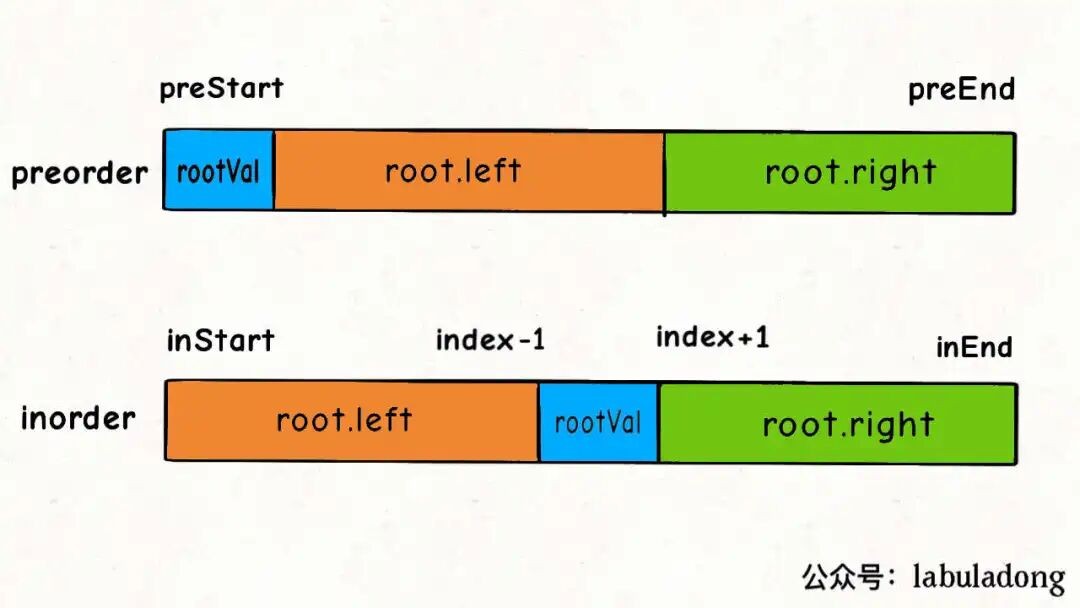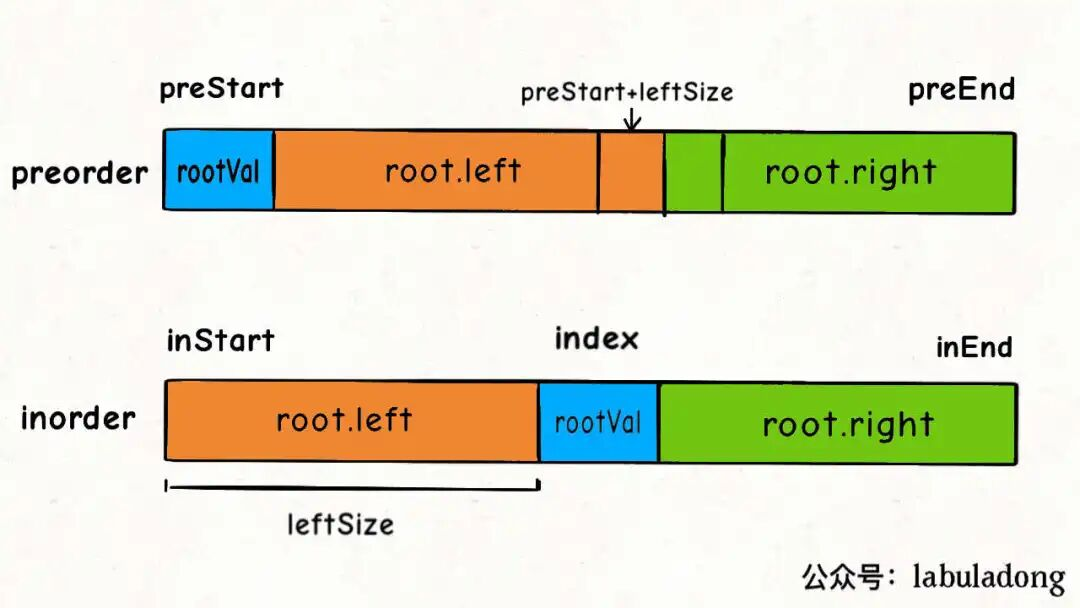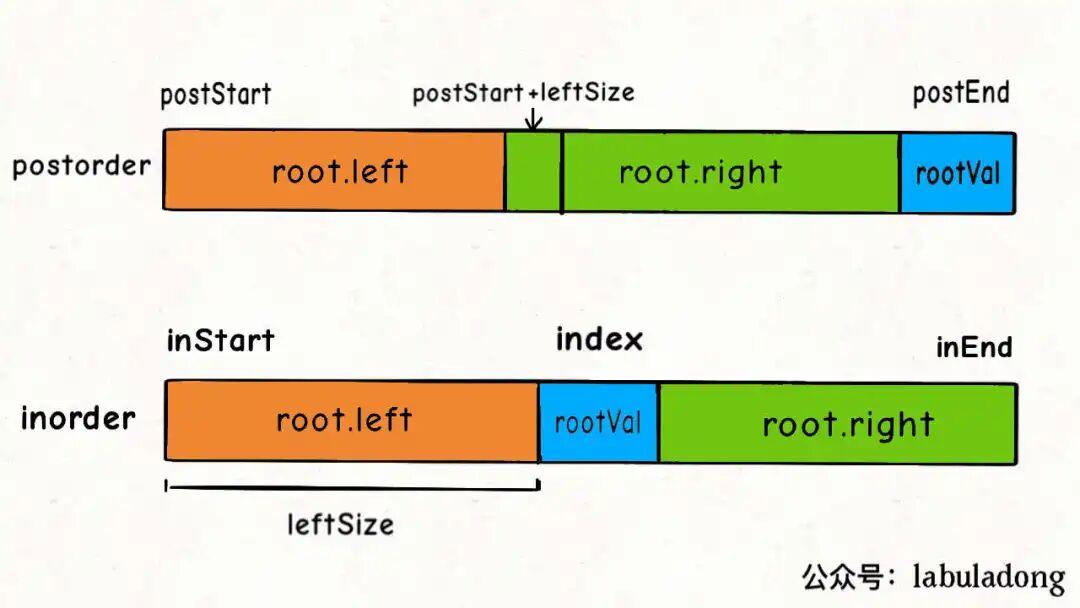## 经典二叉树算法题分析

``````/* 二叉树遍历框架 */
void traverse(TreeNode root) {
// 前序遍历
traverse(root.left)
// 中序遍历
traverse(root.right)
// 后序遍历
}
``````

## 算法实践

### 翻转二叉树

``````// 将整棵树的节点翻转
TreeNode invertTree(TreeNode root) {
// base case
if (root == null) {
return null;
}

/**** 前序遍历位置 ****/
// root 节点需要交换它的左右子节点
TreeNode tmp = root.left;
root.left = root.right;
root.right = tmp;

// 让左右子节点继续翻转它们的子节点
invertTree(root.left);
invertTree(root.right);

return root;
}

``````

### 填充二叉树节点的右侧指针

(leetcode 116)给定一个 完美二叉树 ，其所有叶子节点都在同一层，每个父节点都有两个子节点。二叉树定义如下：

struct Node { int val; Node *left; Node *right; Node *next; } 填充它的每个 next 指针，让这个指针指向其下一个右侧节点。如果找不到下一个右侧节点，则将 next 指针设置为 NULL。

``````Node connect(Node root) {
if (root == null || root.left == null) {
return root;
}

root.left.next = root.right;

connect(root.left);
connect(root.right);

return root;
}
``````

``````// 主函数
Node connect(Node root) {
if (root == null) return null;
connectTwoNode(root.left, root.right);
return root;
}

// 定义：输入两个节点，将它俩连接起来
void connectTwoNode(Node node1, Node node2) {
if (node1 == null || node2 == null) {
return;
}
/**** 前序遍历位置 ****/
// 将传入的两个节点连接
node1.next = node2;

// 连接相同父节点的两个子节点
connectTwoNode(node1.left, node1.right);
connectTwoNode(node2.left, node2.right);
// 连接跨越父节点的两个子节点
connectTwoNode(node1.right, node2.left);
}
``````

### 将二叉树展开为链表(leetcode 114)

``````void flatten(TreeNode root);
``````

`flatten`函数输入一个节点`root`，那么以`root`为根的二叉树就会被拉平为一条链表

1、将`root`的左子树和右子树拉平。

2、将`root`的右子树接到左子树下方，然后将整个左子树作为右子树。

``````// 定义：将以 root 为根的树拉平为链表
void flatten(TreeNode root) {
// base case
if (root == null) return;

flatten(root.left);
flatten(root.right);

/**** 后序遍历位置 ****/
// 1、左右子树已经被拉平成一条链表
TreeNode left = root.left;
TreeNode right = root.right;

// 2、将左子树作为右子树
root.left = null;
root.right = left;

// 3、将原先的右子树接到当前右子树的末端
TreeNode p = root;
while (p.right != null) {
p = p.right;
}
p.right = right;
}
``````

## 构造最大二叉树（leetcode 654)

``````/* 主函数 */
TreeNode constructMaximumBinaryTree(int[] nums) {
return build(nums, 0, nums.length - 1);
}

/* 将 nums[lo..hi] 构造成符合条件的树，返回根节点 */
TreeNode build(int[] nums, int lo, int hi) {
// base case
if (lo > hi) {
return null;
}

// 找到数组中的最大值和对应的索引
int index = -1, maxVal = Integer.MIN_VALUE;
for (int i = lo; i <= hi; i++) {
if (maxVal < nums[i]) {
index = i;
maxVal = nums[i];
}
}

TreeNode root = new TreeNode(maxVal);
// 递归调用构造左右子树
root.left = build(nums, lo, index - 1);
root.right = build(nums, index + 1, hi);

return root;
}

``````

## 通过前序和中序遍历结果构造二叉树``````/* 主函数 */
TreeNode buildTree(int[] preorder, int[] inorder) {
return build(preorder, 0, preorder.length - 1,
inorder, 0, inorder.length - 1);
}

TreeNode build(int[] preorder, int preStart, int preEnd,
int[] inorder, int inStart, int inEnd) {

if (preStart > preEnd) {
return null;
}

// root 节点对应的值就是前序遍历数组的第一个元素
int rootVal = preorder[preStart];
// rootVal 在中序遍历数组中的索引
int index = 0;
for (int i = inStart; i <= inEnd; i++) {
if (inorder[i] == rootVal) {
index = i;
break;
}
}

int leftSize = index - inStart;

// 先构造出当前根节点
TreeNode root = new TreeNode(rootVal);
// 递归构造左右子树
root.left = build(preorder, preStart + 1, preStart + leftSize,
inorder, inStart, index - 1);

root.right = build(preorder, preStart + leftSize + 1, preEnd,
inorder, index + 1, inEnd);
return root;
}
``````

## 通过后序和中序遍历结果构造二叉树``````TreeNode build(int[] inorder, int inStart, int inEnd,
int[] postorder, int postStart, int postEnd) {

if (inStart > inEnd) {
return null;
}
// root 节点对应的值就是后序遍历数组的最后一个元素
int rootVal = postorder[postEnd];
// rootVal 在中序遍历数组中的索引
int index = 0;
for (int i = inStart; i <= inEnd; i++) {
if (inorder[i] == rootVal) {
index = i;
break;
}
}
// 左子树的节点个数
int leftSize = index - inStart;
TreeNode root = new TreeNode(rootVal);
// 递归构造左右子树
root.left = build(inorder, inStart, index - 1,
postorder, postStart, postStart + leftSize - 1);

root.right = build(inorder, index + 1, inEnd,
postorder, postStart + leftSize, postEnd - 1);
return root;
}
``````

## 寻找重复子树（leetcode 652）

1、以我为根的这棵二叉树（子树）长啥样

2、以其他节点为根的子树都长啥样

``````void traverse(TreeNode root) {
traverse(root.left);
traverse(root.right);
/* 解法代码的位置 */
}
``````

``````String traverse(TreeNode root) {
// 对于空节点，可以用一个特殊字符表示
if (root == null) {
return "#";
}
// 将左右子树序列化成字符串
String left = traverse(root.left);
String right = traverse(root.right);
/* 后序遍历代码位置 */
// 左右子树加上自己，就是以自己为根的二叉树序列化结果
String subTree = left + "," + right + "," + root.val;
return subTree;
}
``````

``````// 记录所有子树
HashSet<String> memo = new HashSet<>();
// 记录重复的子树根节点

String traverse(TreeNode root) {
if (root == null) {
return "#";
}

String left = traverse(root.left);
String right = traverse(root.right);

String subTree = left + "," + right+ "," + root.val;

if (memo.contains(subTree)) {
// 有人和我重复，把自己加入结果列表
} else {
// 暂时没人跟我重复，把自己加入集合
}
return subTree;
}

``````

``````// 记录所有子树以及出现的次数
HashMap<String, Integer> memo = new HashMap<>();
// 记录重复的子树根节点

/* 主函数 */
List<TreeNode> findDuplicateSubtrees(TreeNode root) {
traverse(root);
return res;
}

/* 辅助函数 */
String traverse(TreeNode root) {
if (root == null) {
return "#";
}

String left = traverse(root.left);
String right = traverse(root.right);

String subTree = left + "," + right+ "," + root.val;

int freq = memo.getOrDefault(subTree, 0);
// 多次重复也只会被加入结果集一次
if (freq == 1) {